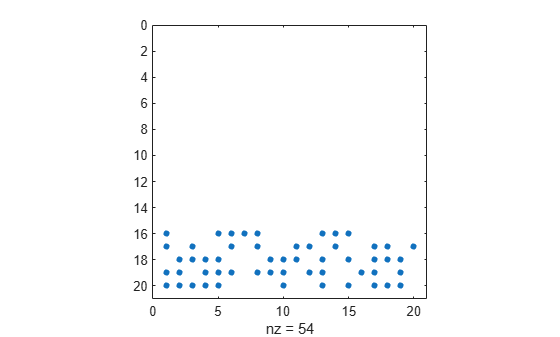# spalloc

Allocate space for sparse matrix

## Syntax

``S = spalloc(m,n,nz)``

## Description

example

````S = spalloc(m,n,nz)` creates an all-zero sparse matrix `S` of size `m`-by-`n` with room to hold `nz` nonzero elements, where `nz >= 1`.```

## Examples

collapse all

Use `spalloc` to initialize a 10-by-10 all-zero sparse matrix with room for up to 20 nonzero elements.

`S = spalloc(10,10,20);`

Define several elements in the matrix.

`S(1:3,1:3) = magic(3)`
```S = (1,1) 8 (2,1) 3 (3,1) 4 (1,2) 1 (2,2) 5 (3,2) 9 (1,3) 6 (2,3) 7 (3,3) 2 ```

Show the number of nonzero elements in the matrix.

`n1 = nnz(S)`
```n1 = 9 ```

Show the amount of storage allocated for nonzero matrix elements.

`n2 = nzmax(S)`
```n2 = 20 ```

Use `spalloc` to initialize a 20-by-20 all-zero sparse matrix with space for 100 nonzero elements.

```n = 20; S = spalloc(n,n,5*n);```

Then use a `for` loop to fill in the columns of `S` one at a time with an average of at most five nonzero elements per column.

```for j = 1:n S(:,j) = [zeros(n-5,1); round(rand(5,1))]; end```

Plot the sparsity pattern of matrix `S`. The dots represent the nonzero elements.

`spy(S)`Show the number of nonzero elements in the matrix.

`n1 = nnz(S)`
```n1 = 54 ```

Show the amount of storage allocated for nonzero matrix elements.

`n2 = nzmax(S)`
```n2 = 100 ```

## Input Arguments

collapse all

Number of matrix rows, specified as a nonnegative integer.

Data Types: `single` | `double` | `int8` | `int16` | `int32` | `int64` | `uint8` | `uint16` | `uint32` | `uint64` | `logical`

Number of matrix columns, specified as a nonnegative integer.

Data Types: `single` | `double` | `int8` | `int16` | `int32` | `int64` | `uint8` | `uint16` | `uint32` | `uint64` | `logical`

Storage allocation for nonzero elements, specified as a nonnegative integer. If you specify a value of 0 for `nz`, then `spalloc` instead sets the value of `nz` to 1.

Data Types: `single` | `double` | `int8` | `int16` | `int32` | `int64` | `uint8` | `uint16` | `uint32` | `uint64` | `logical`

## Limitations

• Both matrix dimensions, `m` and `n`, must be smaller than `2^31-1` on 32-bit platforms, or `2^48-1` on 64-bit platforms.

## Tips

• When you assign several times into a matrix you created with `spalloc`, the preallocated memory can prevent repeated reallocations. However, assigning into a sparse matrix is still a relatively expensive operation, which should usually be avoided if it can easily be replaced by one of the following:

## Version History

Introduced before R2006a